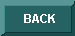HYPERG V 1.0List of functions• AddParam - add a parameter to a [basic] hypergeometric series in standard notation
• BaseSplit - Rules to handle any factorial expression (Gamma function, and Rising Factorial)
• CheckRec - tests if a term satisfies a recurrence relation
• Ext1 & Ext2 - Rules to handle any factorial expression (Gamma function, and Rising Factorial)
• FirstTerms - extracts first terms of a summation
• GenQRec - generate a q-recurrence
• GenRec - generate a recurrence
• Gosper - Gosper's algorithm for summation
• Homog - Homogenizes a recurrence equation
• HypContig - Contiguous formula in form of rules
• HypContigPrint - Print a contiguous formula in form of an equation
• HypConverg - test if is a convergent hypergeometric series
• HypDiff - Differentiation of a hypergeometric series
• HypEval - Rule that transforms a HYP[] into a Sum()
• HypOrder - Order parameters of a hypergeometric series
• HypPerm[Up/Low/Both] - Rules for permuting parameters in [basic] hypergeometric series
• HypSimplify - Apply simplification rules to [basic] hypergeometric series
• HypSolQRec - linear q-recurrence equation solver - hypergeometric solutions
• HypSolRec - linear recurrence equation solver - hypergeometric solutions
• HypSum - Summation formula in form of rules
• HypSumList - gives a list of applicable summation formulas
• HypSumPrint - Print a summation formula in form of an equation
• HypToRec - Zeilberger's algorithm
• HypToVHyp - convert hypergeometric series into hypergeometric series in very-well-poised order
• HypTransf - Transformation formula in form of rules
• HypTransList - gives a list of applicable transformation formulas
• HypTransfPrint - Print a transformation formula in form of an equation
• HypType - Print the type of a hypergeometric series
• HypergToRec - return a linear first-order homogeneous recurrence satisfied by a hypergeometric term
• Inv - Rules to handle any factorial expression (Gamma function, and Rising Factorial)
• IsHYP - test if is a hypergeometric series
• IsHomog - tests if a recurrence equation is homogeneous
• IsHyperg - test if is a hypergeometric term
• IsQBIN - test if is a q-binomial coefficient
• IsQHYP - test if is a basic hypergeometric series
• IsQRF - test if is a q-rising factorial
• IsRF - test if is a rising factorial
• IsVHYP - test if is a hypergeometric series in very-well-poised order
• IsWHYP - test if is a basic hypergeometric series in very-well-poised order
• Lim - compute formal limit of hypergeometric expressions
• Linear1 & Linear2 - Rules to handle any factorial expression (Gamma function, and Rising Factorial)
• MapList & MapApply - Functions for controlled application of rules and functions
• Neg1 & Neg2 - Rules to handle any factorial expression (Gamma function, and Rising Factorial)
• PolySolQRec - linear q-recurrence equation solver - polynomial solutions
• PolySolRec - linear recurrence equation solver - polynomial solutions
• Prove - Zeilberger's algorith (automatic proof)
• QBinEval - Rule that evals q-binomial coefficients
• QHypEval - Rule that transforms a QHYP[] into a Sum()
• QHypOrder - Order parameters of a basic hypergeometric series
• QHypToWHyp - convert basic hypergeometric series into basic hypergeometric series in very-well-poised order
• QHypType - Print the type of a basic hypergeometric series
• QRfEval - Rule that evals q-rising factorials
• RatioSolQRec - linear q-recurrence equation solver - rational solutions
• RatioSolRec - linear recurrence equation solver - rational solutions
• RecOrder - computes the order of a recurrence equation
• RfEval - Rule that evals rising factorials
• ShiftRec - shifts a recurrence equation
• SimplifyRec - simplifies a recurrence equation
• Split - Rules to handle any factorial expression (Gamma function, and Rising Factorial)
• SubsRec - substitutes (in a recurrence) the sequence by a term
• SumToHyp - convert summations into hypergeometric series
• SumToRec - Zeilberger's algorithm
• SummandToRec - Zeilberger's algorithm
• Time - gives time information
• Trans - Rules to handle any factorial expression (Gamma function, and Rising Factorial)
• VHypToHyp - convert hypergeometric series in very-well-poised order into hypergeometric series in standard notation
• WHypToQHyp - convert basic hypergeometric series in very-well-poised order into basic hypergeometric series in standard notation